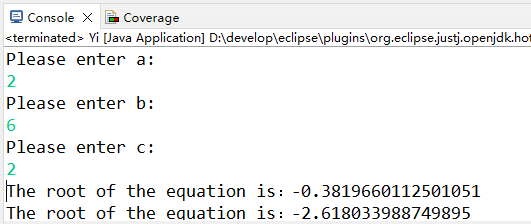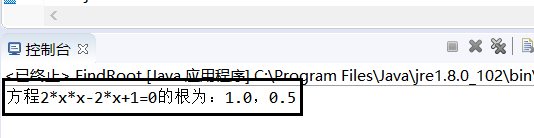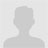• java计算一元二次方程的根
千次阅读
2022-02-27 09:46:46

使用系统：Windows10；

使用JDK：Java SE Development Kit 17.0.2；

使用开发环境：eclipse。

一、代码

package Hello;
import java.util.Scanner;

public class Yi{
public static void main(String[] args) {

double a,b,c;
while(true) {
Scanner sc = new Scanner(System.in);
a=sc.nextDouble();
Scanner sc1 = new Scanner(System.in);
b=sc1.nextDouble();
Scanner sc2 = new Scanner(System.in);
c=sc2.nextDouble();
if(b*b-4*a*c<0) {
System.out.println("The equation has no solution");
}
else {
double X1=(-b+Math.sqrt(b*b-4*a*c))*1/(2*a);
double X2=(-b-Math.sqrt(b*b-4*a*c))*1/(2*a);
System.out.println("The root of the equation is："+X1);
System.out.println("The root of the equation is："+X2);
break;
}
}
}
}

二、执行结果三、粗浅理解

众所周知，一元二次方程有四种解法：1、直接开平方法；2、配方法；3、公式法；4、因式分解法。而我采用最简单的公式法进行求根。

首先创建一个Hello名字的包，再导入java.util包下的Scanner类，import java.util.Scanner的作用就是用来构建Scanner对象。

然后声明一个公共的类的作用范围，且类名要和文件名相同。然后声明程序访问权限，方法为静态的，不依赖类的对象的，不需要返回值的main方法，参数String[] args是一个字符数组，接受来自程序执行时传进的参数。

再然后定义三个双精度浮点型局部变量a,b,c，利用Sanner sc = new Scanner(System.in);得到一个标准的输入流，并创建一个新的基于输入流的对象，差不多的作用是获取控制台的输入，然后并用输入的内容给a,b,c分别赋值。

最后在while循环里嵌套一个if判断语句，首先判断是否b²-4ac的值是否会小于0，小于就是无解。然后根据求根公式x=[-b±(b²-4ac)^(1/2)]/(2a)，计算一元二次方程的两个解分别赋值给x1和x2,最后在控制台输出两个根的值，利用break语句跳出while循环。此时Java实现一元二次方程求根问题解决。

更多相关内容
• b2-4ac称为一元二次方程的判别式，如果它是正值，那么方程有两个实数；如果他为0，方程只有一个；如果它是负值，方程无实数
• 一元二次方程根java,servlet,利用bean类bao装数据
• class FindRoot{ //计算一元二次方程 static double[] root(double a, double b, double c) throws Exception { double x[]=new double; if (a== 0) { throw new Exception("a 不能为零."); } ...
class FindRoot{ //计算一元二次方程的根
static double[] root(double a, double b, double c) throws Exception
{
double x[]=new double;
if (a== 0)
{
throw new Exception("a 不能为零.");
}
else{
double disc = (b*b) - 4*a*c;
if (disc < 0)
throw new Exception("b*b-4ac值不能小于零.");

x=(-b + Math.sqrt(disc)) / (2*a);
x=(-b - Math.sqrt(disc)) / (2*a) ;
return x;
}
}
public static void main(String arg[]) {
try {
double x[] = root(2.0,-3.0,1);	//a,b,c
System.out.println("方程2*x*x-3*x+1=0的根为：" +x+"，"+x);
}
catch(Exception e) { System.out.println(e); }
}
}展开全文• double k;... System.out.println("所要求解的一元二次方程为："+ "a*Y*Y+b*Y+c=0"); System.out.println("a,b,c为常数，Y为自变量，请依次输入a,b,c的值"); Scanner in= new Scanner(System.in);
• java一元二次方程关注:168答案:2mip版解决时间 2021-02-03 01:41提问者青春统帅2021-02-02 10:42out;+k);if(p&gt.in);方程只有一个跟"System.nextDouble()import java.println(&quot.sqrt(p))/.out....

java求一元二次方程的根

关注:168  答案:2  mip版

解决时间 2021-02-03 01:41提问者青春统帅

2021-02-02 10:42

out;+k);

if(p&gt.in);方程只有一个跟"

System.nextDouble()import java.println(&quot.sqrt(p))/.out.println ("

if (a;(2*a).util.nextDouble();

}

}

else{

System;

System.out.println("=0){

if(p>

double b=sc;x2="0){

double x1=(-b+Math;无解"

double c=sc.out;

double a=sc;

public class a1{

public void main(String args[]){

Scanner sc=new Scanner(System;

double x2=(-b-Math;(2*a).println("x1="

System;+x1)!=0)

{

double p=b*b-4*a*c;);+x2).nextDouble().sqrt(p))/(2*a).*;

}

else{

double k=-b/

运行为什么有这个错误？

最佳答案二级知识专家戒烟戒酒戒粗口

2021-02-02 12:12

public void main(String args[]){

这句应改为

public static void main(String[] args)

{

养成好习惯！

全部回答1楼假装安慰

2021-02-02 13:42

程序中您自己写的那个 sqrt 方法，返回值都是 0，那怎么行呢。。

开平方的话直接用 math.sqrt()

修改如下：

import java.util.scanner;

import java.lang.math;

public class shiyan {

public static void main(string[] args) {

double s=0,x1,x2;

system.out.println("输入系数 a b c: ");

scanner in=new scanner(system.in);

double a=in.nextint();

double b=in.nextint();

double c=in.nextint();

s = b*b-4*a*c;

if(a==0)

{

system.out.println("二次方程系数a不能为0！");

return;

}

else if(s==0)

{

x1 = -b/(2*a);

system.out.println("x1="+x1);

return;

}

else if(s>0)

{

double t = math.sqrt(s);

x1 = (-b+t)/(2*a);

x2 = (-b-t)/(2*a);

system.out.println("x1="+x1);

system.out.println("x2="+x2);

return;

}

else if(s<0)

{

system.out.println("该一元二次方程没有实根");

return;

}

}

}

我要举报

如以上问答内容为低俗/色情/暴力/不良/侵权的信息，可以点下面链接进行举报，我们会做出相应处理，感谢你的支持！

→点此我要举报以上信息！←

推荐资讯

大家都在看

展开全文• 我们知道，一元二次方程根的判别式公式如下： 案例代码： import java. util. Scanner; public class EquationRoot{ public static void main( String[ ] args){ double a, b, c; double root; Scanner ...

基本知识：

我们知道，一元二次方程根的判别式公式如下：接下来，我将使用两个案例：案例一是只计算正根的案例，案例二是对的 △ 的大小进行判断根据情况计算根的值案例。

案例一的代码：

import java. util. Scanner;
public class EquationRoot{
public static void main(String[] args){
double a, b, c;
double root;
Scanner input = new Scanner(System.in);
System.out. println("请输入a, b,c的值:");
a = input.nextDouble();
b = input.nextDouble();
c = input.nextDouble( );
root = (-b + Math.sqrt(b * b - 4 * a * c)) / (2 * a);
System.out.println("方程的一个根是:" + root);
}
}



案例一的效果图：案例二的代码：

import java.util.Scanner;
public class Caculateeeeeeee {
public static void main(String[] args) {
int a,b,c;
double x1,x2;
Scanner inputScanner=new Scanner(System.in);
System.out.println("请依次输入a,b,c");
a = inputScanner.nextInt();
b = inputScanner.nextInt();
c = inputScanner.nextInt();
x1 = ((-b)+Math.sqrt(b*b-4*a*c))/(2*a);
x2 = ((-b)-Math.sqrt(b*b-4*a*c))/(2*a);
if(b*b-4*a*c<0) {
System.out.println("方程无实根");
}
else {
System.out.println("方程有实根X1为："+ x1 +"   X2为：" + x2);
}
}
}


案例二的效果图：展开全文• 【提示】要考虑各种情形:一是 a 为0的情形,方程根为-c/......(理论考试重点内容实践) 一元二次方程白盒测试要求 1) 自备 C 语言程序(可从 C 语言程序设计的教科书上摘录若干典型程序,其他 C++、C#、Java 程序均可);...
• //创建一个test包import java.util.Scanner;//导入java.util包中的Scanner类(使用...//导入java.math包中的BigDecimal(用来对超过16位有效位的数进行精确的运算)//对一元二次方程中各系数的不同情况作讨论，列出不同...
• public class TestMath { public static void main(String[] args) { ... System.out.println("计算一元二次方程"); Scanner sc=new Scanner(System.in); System.out.print("请输入a的值：")...
• 【提示】要考虑各种情形:一是 a 为0的情形,方程根为-c/......Java 复习题汇总 编程题 1、编程序,输入 a,b,c,求方程 package 一元二次方程 2; import java.util.Scanner; public class 一元二次方程 2 { public ...
• import java.util.Scanner; public class SolveEquation { public static void main(String[] args) { //输出部分 double a,b,c,delta,x1,x2; Scanner sc=new Scanner(System.in); //输出部分 Sys...
• Java一元二次方程（实验三） Package和import语句的使用 编写Java应用程序求方程。使用package语句将方程的属性即计算根的方法封装在一个有包名的类中，包名是tom.jiafei，类的名字是SquareEquation。编写...
• 今天学习了利用java求解一元二次方程。 从面向对象的角度去解决这个问题。 需要两个对象，即类 求类（EqualRoots） 和 测试类（EqualRootsTest） 类的设计 成员方法 求方法（EqualRoots，public） ...
• } private double calculateValidDisk() { if (a == 0) { throw new EquationException("不是二次方程", EquationException.NONE_EQUATION); } double disk = b * b - 4 * a * c; if (disk ) { throw new ...
• 一元二次方程根Java源程序代码》由会员分享，可在线阅读，更多相关《一元二次方程根Java源程序代码(3页珍藏版)》请在人人文库网上搜索。1、求解一元二次方程ax2+bx+c=0的实和复根，Test类是主类class ...
• Java编写程序，求一元二次方程ax²+bx+c=0的（系数在程序中给定），并输出。
• 题目描述 编写程序用于计算一元二次方程的实。要求定义Equation类以表示一元二次方程，包括：方程系数、实的个数与类型、每个实的值、获得方程的字符串表达方式的方法、求解的方法、输出方法。再定义Main类...开发语言
• 一元二次方程ax2+bx+c=0，输入a,b,c三个系数，求解方程，结果有三种情况：两个实数、一个.../*** 计算一元二次方程* @author TongQiang**/public class Equation {double a,b,c; //方程的系数double x1,x2; /...
• 计算一元二次方程的两个实数 import java.util.Scanner; public class Psg { public static void main(String[] args) { Scanner reader = new Scanner(System.in); System.out.println(“请输入方程的系数a、b、c...
• 可以用eclipse直接打开。 文件说明：calculator.java为编写的一元二次方程组代码，还有两个为junit测试代码。
• ## Java求一元二次方程的根

万次阅读 多人点赞 2018-10-29 22:45:01
一元二次方程。 【问题描述】编写程序，从键盘输入三个系数ax2+bx+c=0，计算方程的解并输出。需要考虑方程有虚根、方程有实方程是一元一次方程、没有等。输出方程的解并保留6位小数。 import java....
• 代码：import java.awt.*;import java.awt.event.*;import javax.swing.*;public class EquationFrame extends JFrame implements ActionListener {SquareEquation equation;JTextField textA, textB, textC;...
• 利用如下方法计算一元二次方程，可以设计一个图形界面输入A,B,C值，点击“求解”按钮进行计算，结果显示在一个标签中。 注意代码的异常处理。
• 满意答案//如一元二次方程:ax*x+bx+c=0,要从控制台输入三个元素a,b,cimport java.util.*;public class Geng{public static void main(String[] args){Scanner input = new Scanner(System.in);double x1,x2;//定义...
• ## java求一元二次方程:ax2+bx+c=0的根

千次阅读 多人点赞 2019-11-28 01:23:18
一元二次方程:ax2+bx+c=0的，a、b、c由键盘输入。
• import java.util.Scanner; public class HelloJava{ public static void main(String[] args) { double a,b,c; while(true) { System.out.println("Please enter a:"); Scanner sc = new Scanner(System....
• 一元二次方程根Java源程序代码.doc (3页) 本资源提供全文预览，点击全文预览即可全文预览,如果喜欢文档就下载吧，查找使用更方便哦！9.90 积分﻿//求解一元二次方程ax^2+bx+c=0的实和复根，Test类是主类class ...
• 前言 这周一直比较忙，所以一直没有学习新的东西，所以决定本周的博客主要巩固一下课堂知识。 MVC模式 M（模型：Model）：用于储存数据的对象。 V（视图：View）：向控制器提交所...实例（计算一元二次方程） Modservlet mvc jsp
• 通过java实现输入系数求一元二次方程根，学习Scanner()、split()和Substring()函数-附件资源...